Electromechanical Indicating Type Instruments - 2

# Electromechanical Indicating Type Instruments - 2 | Electrical Engineering SSC JE (Technical) - Electrical Engineering (EE)

 1 Crore+ students have signed up on EduRev. Have you?

Moving Iron Instruments
These instruments are widely used in laboratories and switchboards at commercial frequencies because these are cheaper in cost, robust in construction and can be manufactured with required accuracy.

They are two general types of such in struments i.e. attraction type instruments operate on the principle of attraction of a single piece so soft iron into a magnetic field and repulsion type in struments operate on the principle of repulsion of two adjacent iron pieces magnetised by the same magnetic field. Repulsion type instruments are more sensitive as in these instruments large operating torque is developed by having tow iron elements positioned close together inside the field coil where the magnetizing effect is maximum. Since instantaneous deflecting torque is proportional to the square of the instantaneous current, hence when the instrument is connected in an ac circuit it will indicate the rms value of current or voltage.

The deflecting torque of moving iron instrument is given by equation.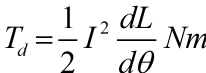The errors usually occurring in moving iron instruments are

(i) friction (ii) temperature (iii) stray magnetic field (iv) hysteresis (v) frequency and (vi) waveform.

These instruments are simple and robust in construction, cheaper in cost, possess high operating torque, capable of withstanding overload momentarily, capable of giving an accuracy within the limits of both precision and industrial grades and can be used in dc as well as in ac circuits. The drawbacks of moving iron instruments are non-uniform scale, higher power consumption for low voltage range, errors are caused due to hysteresis and stray magnetic field, errors are caused due to change in frequency and waveform in ac measurements and there is a difference between dc and ac calibration because of inductance effect of the instrument and eddy currents on ac.

The moving iron instruments, owing to their inherent advantages, have become most widely used instruments in ac circuits. In addition, they are largely used in dc circuits in cases wher the superior accuracy of moving coil instruments is not an essential requirement.

Moving coil instruments.
There are two types of moving coil instrument magnet type and dynamometer type. Permanent magnet moving coil instrument (PMMC) is the most accurate and useful for dc measurement. Dynamometer type moving coil instrument can be used both on dc as well ac. Their calibration is the same for both dc as well as ac and so these instruments are very useful as 'transfer instruments'.xc

1. Permanent Magnet Moving Coil (PMMC) Instrument.

The basic operating principle of a permanent magnet moving coil instrument is the same as that of D' Arsonval galvanometer except that there is slight difference from the D' Arsonval galvanometer in construction, which makes it portable and convenient in use. The suspension, Employed in galvanometer, is replaced by hardened steel pivots and the controlling torque is provided by spiral or helical springs in absence so suspension. The springs also conduct the operating current into and out of the moving coil. The mirror and optical system usually employed in D' Arsonval galvanometer are replaced by a pointer attached to the moving system and a fixed scale, calibrated for indicating the quantity under measurement directly. The PMMC instrument have the advantages of low power consumption (say μW to 200μW ) because of small driving torque, no hysteresis loss (former being of copper or aluminum), very effective and reliable eddy current damping high torque-weight ratio resulting in high accuracy (2-5% of full-scale reading), no stray magnetic field effect because of use of intensive polarised or unidirectional field, use of single instrument for measurements of currents and voltages by using shunts and multipliers of different resistances.

The deflecting torque of a PMMC instrument is given by equation and deflection Td =  BilNr Nmθai

2. Dynamometer type instruments
Are very similar to PMMC instruments except that the permanent magnetic field is replaced by a coil (usually two fixed air cored coils are used) which carry the current to be measured (or a definite fraction of it or proportional to the voltage to be measured). The coils are usually air-cored to avoid hysteresis, eddy currents and other error when the instrument is used on ac. The fixed coil is divided into two halves, connected in series with the moving coil and placed together and parallel to each other so as to provide a fairly uniform field within the range of the movement of the moving coil. However, the space between the two halves of the fixed coil must be sufficient enough to allow the passage of the moving coil shaft.

Though these instruments can be used both, for dc and ac measurements but most of its practical uses are in ac as these instruments have higher cost, higher power consumption, lower torque-weight ratio, nonuniform scale and other several draw-backs in comparison to PMMC instruments.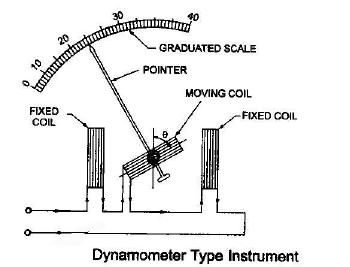Deflecting torque of a dynamometer instrument is given by equation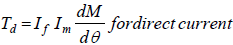and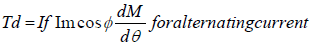where If and Im are current in fixed coil and moving coil respectively.

The errors, that usually occur in dynamometer type instruments, are

(i) frictional error (ii) temperature error (iii) stray magnetic field error and (iv) frequency errors (reactance error and eddy current error)

These instruments have the drawbacks of

(i) comparatively high power consumption, (ii) nonuniform scale (iii) small torque-weight ratio resulting in considerable friction error (iv) more frictional losses (v) necessity of adequate screening of the movements against stray magnetic fields (vi) higher cost and (vii) very low sensitivity (the sensitivity of dynamometer type voltmeter is 10-30 Ω/V )

PMMC (Permanent Magnet Moving Coil)
Type Instruments: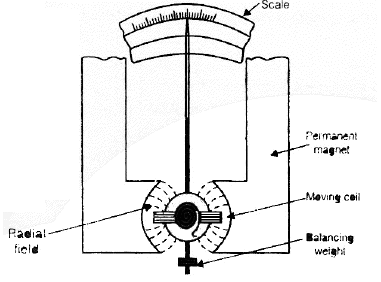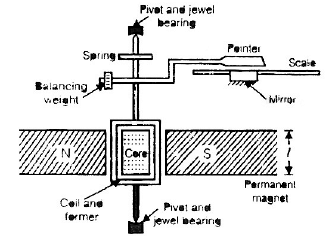Permanent magnet moving coil instrment (PMMC)

• In this instrument, the strong magnetic field produced is provided by the high permeability iron core (stationary).
• Operating field (B) is produced by the permanent magnetic material and it may vary from 0.1 wb/ m2 – 1.0 wb/m2.
• It consist of fixed magnet made up of Al NiCO (Al + Ni + CO).
• Concentric magnetic construction is used to get longer angular movement of the pointer.
• Angular displacement can be over 300º.

• In Voltmeter, PMMC is mouted on meallic frame to provide electromagnetic damping whereas in Ammeter, PMMC is wound on non-magnetic former because the electromagnetic damping is provided by coil of the ammeter shunt.
• The moving coil is sustained by control spring which is made up of “Phospher Bronze” Provides:
⇒ Controlling torque (Restoring torque).
⇒ Lead current in and out of the coil.
• Al-former (Moving) is attached to the spindle is responsible for Eddy current damping.

Note :
⇒The current through the PMMC instrument is range in 20 mA. The large current can damage the moving system of instrument.
⇒ If spring of P.M.M.C. instrument is broken the reading is zero because the current does not flow in coil but in moving coil it shows full scale. Whereas in moving iron type spring is broken, the reading will be beyond full scale.

Expression of torque Produced in PMMC: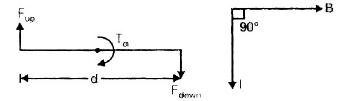Force of conductor = F = NBIl sin 90º = NBIℓ since two equal and opposite forces are produced on MC, so a deflecting torque (Td) is developed,

Td = F x d

= NBIℓ x d

Td = NBIA

Also Td = GI

where, N = No. of turns in coil
B = Magnetic flux density (wb/m2)
I = Current through coil
A = (l × d) = area of c/s of coil
G = (NBA) = Galvanometer constant
Now the restraining torque (controlling torque) provided by spring control is,

Tc = Kθ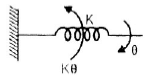At final or null deflection or at steady deflection,

Tc = Td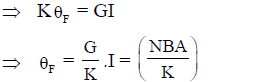(where, θF = deflection produced in instrument)

θ∝ 1 and θ∝ 1/K

Hence, the scale in PMMC is liner
Note:
⇒If spring constant is reduced to half the instruments (PMMC) reading will be doubled.
⇒ Hence with the ageing effect of spring the reading will be more.

Ammeter Shunt in PMMC:
• The basic movement of a d.c. ammeter is a PMMC d'Arsonval galvanometer.
• This is used to raised the current range of ammeter.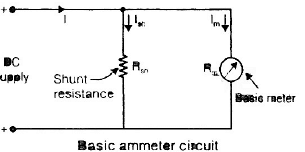Apply current division rule,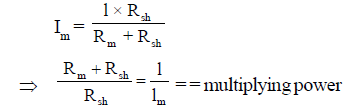where,
Rsh = Shunt resistance ( Ω )
Rm = Internal resistance of coil ( Ω )
lm = Full scale deflection current (A)
l = Current to be measured (A)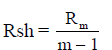The ratio of total current to the current in the movement is called multiplying power (m)

m = I/Im

Properties of Ammeter Shunt:
• Ammeter shunt should have low temperature coefficient, small size and low thermal e.m.f. with copper.
• The copper shunt are not used because of its bulky size and its high resistivity.
• The most commonly used material for D.C. shunt is “Manganin”. Because it has low thermal e.m.f. with copper and low temperature coefficient.
• The resistance of shunts should not vary with time.

Note:
⇒ “Constantan” is a useful material which is used for the construction of a.c. measuring instrument.

PMMC as Voltmeter:
• This has main purpose to increase the voltage range of voltmeter.
• A d'arsonval basic PMMC is converted into a voltmeter by connecting a series resistance (Rsv) with and this resistance is called multiplier resistance (Rsv).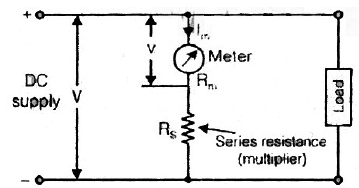By using voltage division rule we get,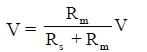where, RS = multiplier resistance ( Ω )
V = extended full range voltage (Volt)
lm = lfs = full scale deflection current
V = voltage across the meter at full defection.

and voltage across the meter movement for current lm,

V = ImRm

Now,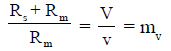where, mv is the multiplying factor for multiplier.
⇒ Resistance of multiplier for voltmeter Rsv = (mv – 1) Rm
⇒ Hence for the measurement of voltage m times the voltage range of instrument, the Rsv should be (m – 1) times the meter resistance.
⇒ Resistance of multiplier for ammeter,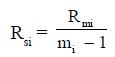Voltmeter sensitivity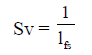lfs ⇒ full scale deflection current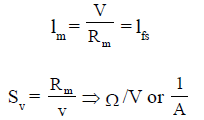Multiplier Resistance in terms of Sensitivity
From figure V = lm R+ lm Rm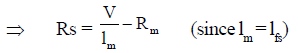Rs = VSv – Rm

Properties of voltmeter series or multipliers:
• Their resistance should not change with time.
• They should be non inductively would for AC meter.
• Variation of resistance w.r.t. temperature should be small and it is eliminated by “swamp resistance”.
• Materials used for multipliers are as,
⇒ For DC application ⇒ Manganin
⇒ For AC application ⇒ Constantan

Moving Iron Type Instrument:
• The most common Ammeters and Voltmeters for laboratory or switch board used at power frequencies are the Moving iron instruments.
• M. I. type instrument may be of attraction type moving iron or repulsion type moving iron.
⇒ Attraction type M. I. instrument is shown below in figure.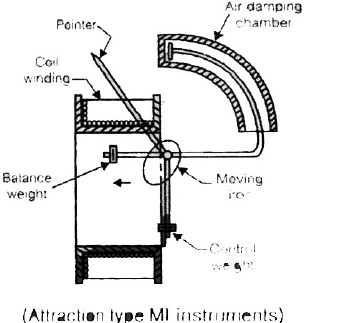• Attraction type consist of movable iron vane attached to the spindle. Iron vane is always attracted towards the centre of fixed coil.
• When the current flows through the coil, a magnetic field is produced and MI moves from weaker field outside the coil to the stronger field inside it or in other words the MI is attracted in.
• The controlling torque (Tc) is provided by springs but gravity control can be used for pannel of instruments.
• Damping is provided by “Air-friction damping” with the help of “Light Al-piston” which moves in a fixed chamber closed at one end as shown in above figure
• The operating magnetic field in MI is very weak so, eddy current damping in not used.
• Range of magnetic field is 0.0062 to 0.0075 wb/ m2.
• If frequency of input is large the eddy current induced in iron vane may distort the main flux or field of the coil. Therefore, frequency of signal in moving iron type instrument is restricted to 0 - 125 Hz.

Note:
⇒ In both type (Attraction or repulsion) of MI instrument, the iron vanes are magnetised in such a way by the current that there is always a force of attraction in the “Attraction type” and repulsion in the “Repulsion type”. Thus we c an say Moving iron type instrument are unpolarised instruments i. e. They are independent of the direction in which the current flows.

So, these “MI instruments” can be used on both AC and DC purposes.

Expression for deflecting torque in MI type: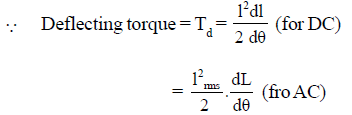where,
L = inductance of coil.
I = current through the coil.
θ = deflection of the pointer.
And control torque,
Tc = k θ
At balance condition or at final deflectional
T= Td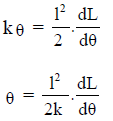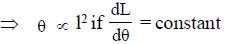⇒ The scale is non-linear or non-uniform because θ ∝ I2

Note:
⇒ Moving iron type instrument measured RMS value of input quantity.
⇒ MI type instruments are less accurate but cheaper.

Electrodynamometer Type instrument: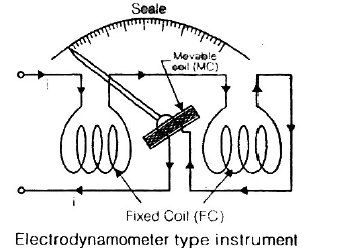• Electordynamometer type instruments consists of two coil, fixed ciol (FC) and Moving ciol (MC).
• Fixed coil is splitted into two part in order to have uniform distribution of flux around moving ciol.
• FC is used to provide operating field.
• MC is a current carrying coil on which deflection torque (Td) is produced.
• For measurement of voltage and current both coils are connected inseeries. Deflection produced in moving coil is same for a.c. and d.c. and is proportional to the r.m.s.value of a.c. Therefore, these are first calibrated on d.c. This calibration is then transferred to the a.c. instrument on alternating current.
• Spring control is used and Control spring is provided for dual purposes as,

(i) To generate spring control torque.
(ii) Provide path for current to the moving coil.

• If spring is broken, controlling torque is zero and Meter reads zero.
• Air-friction damping is used here.

Single-phase Electrodynamometer Power Factor Meter:

• he power factor, by definition is the cosine of the phase angle between voltage and current, and power-factor measurements usually involve the determination of this phase angle.
• The construction of a single-phase electrodynamometer power factor meter is shown in figure below.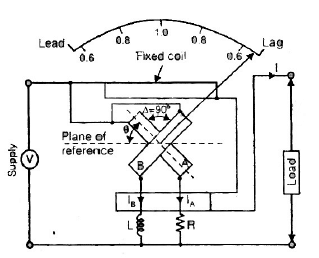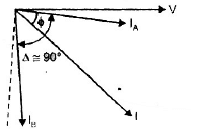.

• This is demonstrated in the operation of the crossed-coil power-factor meter. In this instruments. the moving element consists of two coil, mounted on the same shaft but at right angles to each other. The moving coils rotate in the magnetic field provided by the field coil that carries the line current.
• One coil of the movable element is connected in series with a resistor across the lines and receives its current from the applied potential difference.
• The second coil of the movable element is connected in series with an inductor across the lines. Since no-control springs are used, the balance position of the movable element depends on the resulting torque developed by the two crossed coils.
• When the movable element is in a balance position, the contribution to the total torque by each element must be equal but of opposite sign.
• The developed torque in each coil is a function of the current through the coil and therefore depends on the impedance of the coil circuit.
• The torque is also proportional to the mutual inductance between each part of the crossed coil and the stationary field coil.
• This mutual inductance depends on the angular position of the crossed-coil elements with respect to the position of the stationary fields coil.
• When the movable element is at balance it can be shown that its angular displacement is a function of the phase-angle between line current
• (field coil) and line voltage (crossed coils). The indication of the pointer, which is connected to the movable element is calibrated directly in terms of the phase angle or power factor.

⇒Let us consider the case of a lagging power factor of cosø .
Deflecting torque acting on coil A is,

TA = KVI Mmax cosø sinθ
θ = angular deflection from the plane of reference
Mmax = Maximum value of mutual inductance between the two coils.
This towque say acts in th eclock-wise direction.

Direction torque acting on coil B is;

lB = KVI Mmax cos (90º – ø )sin (90º + θ ) = KVI Mmax sinø cosθ

This torque acts in the anti-clockwise direction.
Hence at equilibrium,
T= TB

or KVI Mmax cosø sinθ = KVI Mmax sinø cosθ

cosø sinθ = sinø cosθ

cosø sinθ - sinø cosθ = 0

sin(ø - θ) = 0

(ø - θ) = 0

ø = θ

Watthour Meter :
• It is not often found in a laboratory but it is widely used for the commercial measurement of electrical energy.
• Figure below shows the elements of a single phase watthour meter.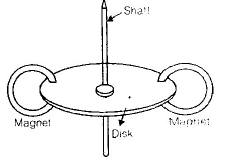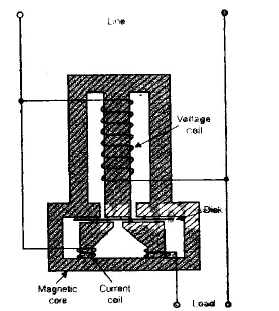• In the figure above, both coils (current coils and voltage coils) are would on a metal frame of special design, poviding two magnetic circuits.
• A light Al-disk is suspended in the air gap of current-coil field, which causes eddly currents to flow in the disk.
• The developed torque is proportional to the Fieldstrength of the voltage coils and eddly currents in the disk. The no. of rotations of the disk is therefore proportional to the energy consumed by the load in a curtain time internal, and is measured in terms of kilowatt hours (kWh).
• Damping of the disk is provided by the two small permanent magnets located opposite each other at the rim of disk.

Instrument Transformers :
Instrument transformers are employed for ac measurements (current, voltage, power and energy) in conjunction with the relevant instruments. Such transformers are of two types- Current (or series) transformer and potential (or parallel) transformers.

Current transformer is employed whenever the current of an ac circuit exceeds the sage current of the measuring instrument (e.g. ammeter or wattmeter or energy meter).

Potential transformer is employed whenever and wherever the voltage of an ac circuit exceeds the voltage of 750 volts as it is not easy to provide adequate insulation on measuring instruments for voltage above 750 volts.

(a) Current Transformers (CTs)
The Primary of the CT, which consists of only one of a few turns, is connected in series with the load circuit and so carries the load current to be measured. The secondary winding consists of many more turns than the primary and have the instrument ( an ammeter or ammeter and current coil of wattmeter) directly connected across it. In case of current transformer as the load impedance or 'burden' on the secondary is very small, so the current transformer operates on short-circuit conditions. Also the current in secondary winding is not governed by the load impedance on the secondary but depends upon the current flowing in the primary winding (i.e. main circuit current.) The amount of power which the CT handles is small. The product of voltage and current on the secondary side when the CT is supplying the instrument with its maximum rated value of current, is known as rated burden and is measured in volt-amperes (VA).

There are two types of errors introduced by CT into a circuit These are

(i) ratio error (ii) phase angel error.
CT ratio and phase angle errors are given by equations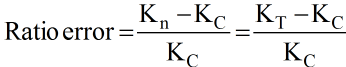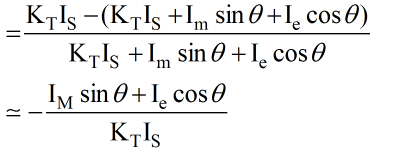Ratio error is taken as + ve when the actual ratio of current transformer is less than nominal ratio. In the above equation of ratio error-ve sign indicates that actual ratio of CT is more than nominal ratio.

The ratio error determines the amount of turns compensation to reduce the error to minimum.

(b) Potential Transformers (PTs)
The potential transformers are used for measurement of high voltages by means of low range voltmeter or for energising the potential coils of wattmeters and energy meters. These are also used for energising relays and other protective equipment. The voltage being measured acts cross the primary winding, which has a large number of turns and connected across the circuit. the secondary winding which has a much smaller number of turns is coupled magnetically through the magnetic circuit to the primary winding. The turn ratio is so adjusted that secondary voltage is applied to the primary.

The errors introduced by PT are less pronounced than those introduced by CTs.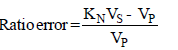where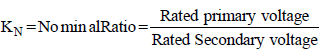Phase angle error,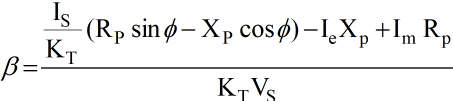The ratio error can be compensated by increasing the turns on secondary winding by the appropriate small amount.

• Instrument transformers (IT) are used to measure AC at generating stations, transformer stations and at transmission lines, in conjunction with AC measuring instruments (Voltmeters, Ammeters, Wattmeters, VARmeters etc.)
• IT performs two important functions and they are :
⇒ They serve to “extend the range” of AC measuring instrument, muxh as the shunt or the multiplier extends the range of DC meter.
⇒ Also, they serve to “isolate” the measuring instrument from the HV power line.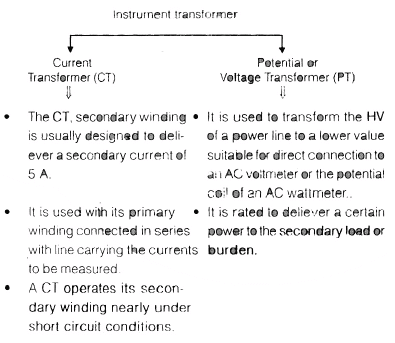The document Electromechanical Indicating Type Instruments - 2 | Electrical Engineering SSC JE (Technical) - Electrical Engineering (EE) is a part of the Electrical Engineering (EE) Course Electrical Engineering SSC JE (Technical).
All you need of Electrical Engineering (EE) at this link: Electrical Engineering (EE)

## Electrical Engineering SSC JE (Technical)

2 videos|75 docs|40 tests

## Electrical Engineering SSC JE (Technical)

2 videos|75 docs|40 tests

Track your progress, build streaks, highlight & save important lessons and more!(Scan QR code)

,

,

,

,

,

,

,

,

,

,

,

,

,

,

,

,

,

,

,

,

,

;